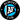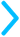## Linear Equation Graphs

Linear equation graphs are always a straight line. And you just need two points to draw a straight line (try it out!), which is the key to drawing the graphs.If you're looking to find the linear equation from a graph, check out our step-by-step guide below. If you're looking to graph a linear equation, scroll down to our linear equation grapher.

### Graph to Linear Equation

There are five easy steps to getting the linear equation from a graph:

1. Find two points: We need two points to figure out our equation. So, we should look for two points that have whole numbers as $x$ and $y$ coordinates.
Let's say this is our graph:
We're going to pick the two blue points, which are $(0, 7)$ and $(-1, 4)$.
2. Get the slope: Next, we need to use our two points to get the slope of the line. Let's remember how to get the slope...

#### Slope Formula

Let's say we have two points: Point 1 with coordinates $\textcolor{#F18F01}{(x_1,y_1)}$ and Point 2 with coordinates $\textcolor{#A14DA0}{(x_2,y_2)}$. The slope is given by the change in $y$ by the change in $x$:$m = \text{slope} = \frac{\textcolor{#F18F01}{y_1} - \textcolor{#A14DA0}{y_2}}{\textcolor{#F18F01}{x_1} - \textcolor{#A14DA0}{x_2}}$
So, with our two points, our slope is:$\frac{\textcolor{#F18F01}{y_1} - \textcolor{#A14DA0}{y_2}}{\textcolor{#F18F01}{x_1} - \textcolor{#A14DA0}{x_2}} = \frac{7-4}{0-(-1)} = \frac{3}{1} = 3$
3. Slope-intercept form: Now, we can plug our slope into slope-intercept form, which is$y = mx + b$
where $m$ is the slope. Plugging in our slope of 3, we get $y = 3x + b$
4. y-intercept: Finally, we need to get our value for $b$. We can do this, by plugging in one of original points. We're going to choose the first point: $(0, 7).$ Plugging in, we get this:$y = 3x + b \implies 7 = 3(0) + b$
If we solve this equation, we get:\begin{aligned} 7 &= 3(0) + b\\ 7 &= 0 + b\\ 7 &= b\end{aligned}
5. Final equation: Now, we can plug our $b$ value in to get our equation:$y = 3x + 7$

### Linear Equation Grapher

There are four easy steps to graphing a linear equation:

1. Slope-intercept form: We need our equation to be in slope-intercept form,$y = mx + b$
where $m$ and $b$ represent numbers. Enter in some numbers for $m$ and $b$.
$y=$$x \> + \>$